Examples from NCERT Book

Class 10
Chapter 10 Class 10 - Light - Reflection and Refraction

## A convex mirror used for rear-view on an automobile has a radius of curvature of 3.00 m. If a bus is located at 5.00 m from this mirror, find the position, nature and size of the image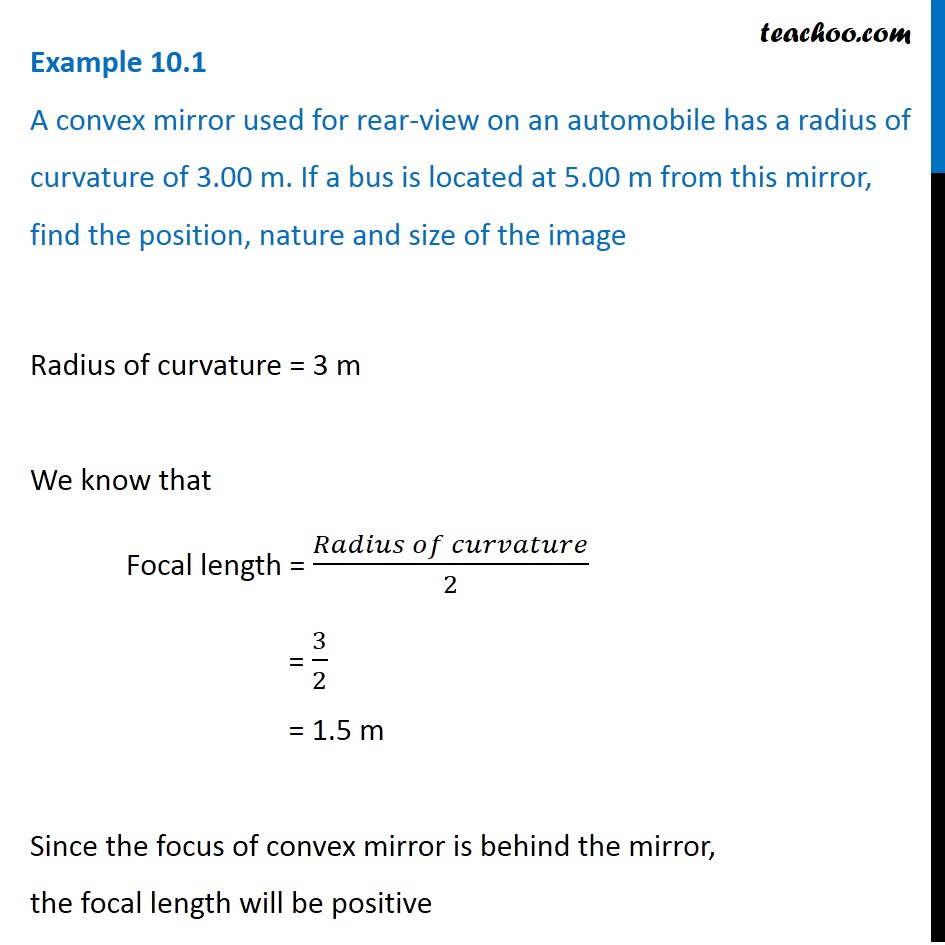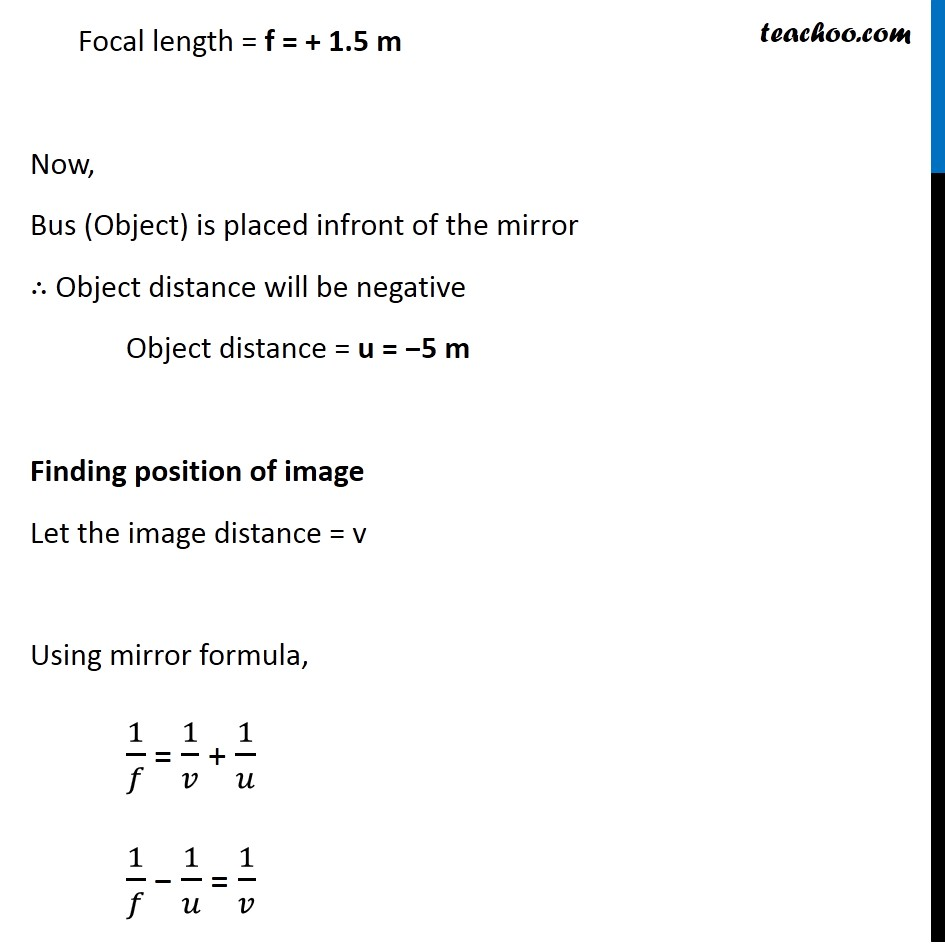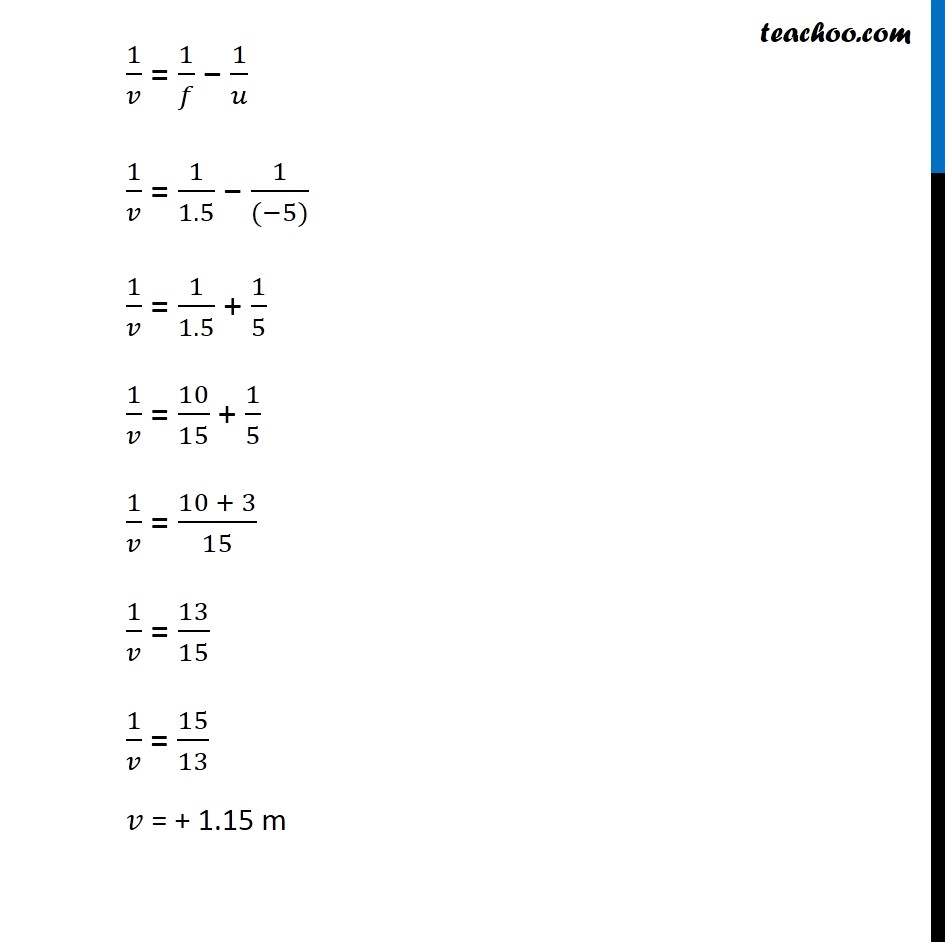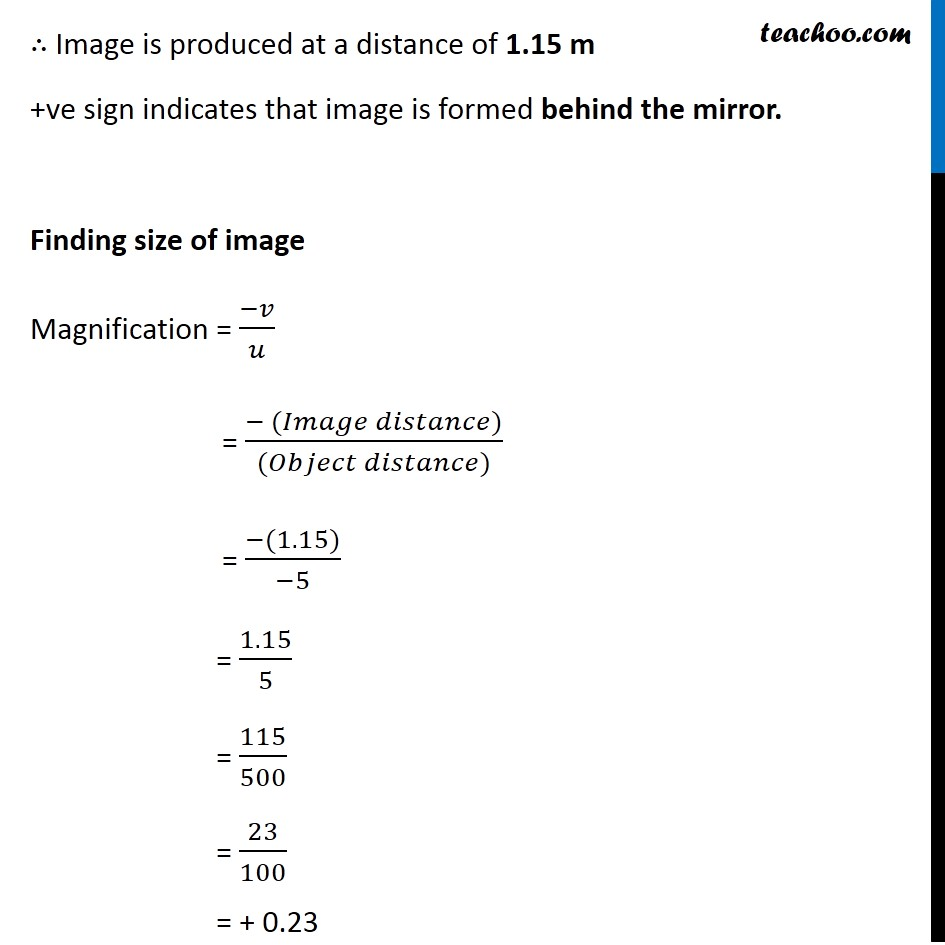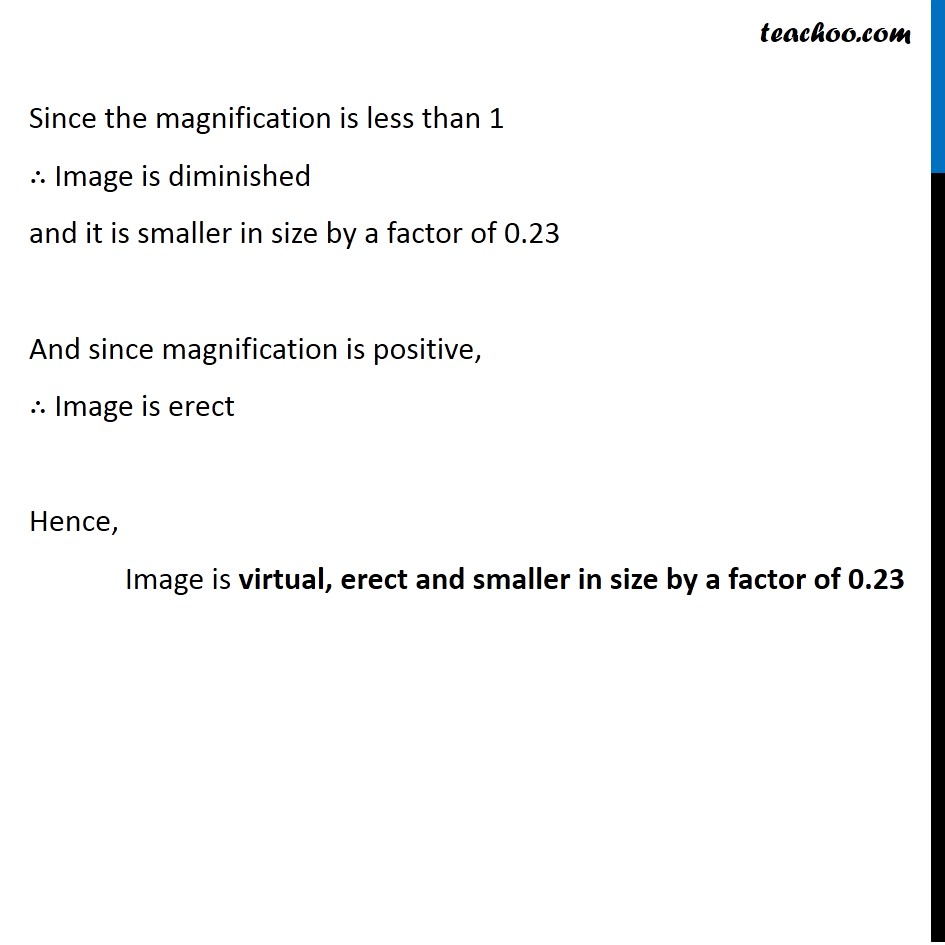Learn in your speed, with individual attention - Teachoo Maths 1-on-1 Class

### Transcript

Example 10.1 A convex mirror used for rear-view on an automobile has a radius of curvature of 3.00 m. If a bus is located at 5.00 m from this mirror, find the position, nature and size of the image Radius of curvature = 3 m We know that Focal length = (𝑅𝑎𝑑𝑖𝑢𝑠 𝑜𝑓 𝑐𝑢𝑟𝑣𝑎𝑡𝑢𝑟𝑒)/2 = 3/2 = 1.5 m Since the focus of convex mirror is behind the mirror, the focal length will be positive Focal length = f = + 1.5 m Now, Bus (Object) is placed infront of the mirror ∴ Object distance will be negative Object distance = u = −5 m Finding position of image Let the image distance = v Using mirror formula, 1/𝑓 = 1/𝑣 + 1/𝑢 1/𝑓 − 1/𝑢 = 1/𝑣 1/𝑣 = 1/𝑓 − 1/𝑢 1/𝑣 = 1/1.5 − 1/((−5)) 1/𝑣 = 1/1.5 + 1/5 1/𝑣 = 10/15 + 1/5 1/𝑣 = (10 + 3)/15 1/𝑣 = 13/15 1/𝑣 = 15/13 𝑣 = + 1.15 m ∴ Image is produced at a distance of 1.15 m +ve sign indicates that image is formed behind the mirror. Finding size of image Magnification = (−𝑣)/𝑢 = (− (𝐼𝑚𝑎𝑔𝑒 𝑑𝑖𝑠𝑡𝑎𝑛𝑐𝑒))/((𝑂𝑏𝑗𝑒𝑐𝑡 𝑑𝑖𝑠𝑡𝑎𝑛𝑐𝑒)) = (−(1.15))/(−5) = 1.15/5 = 115/500 = 23/100 = + 0.23 Since the magnification is less than 1 ∴ Image is diminished and it is smaller in size by a factor of 0.23 And since magnification is positive, ∴ Image is erect Hence, Image is virtual, erect and smaller in size by a factor of 0.23